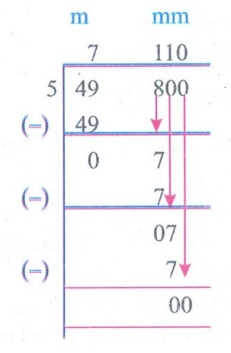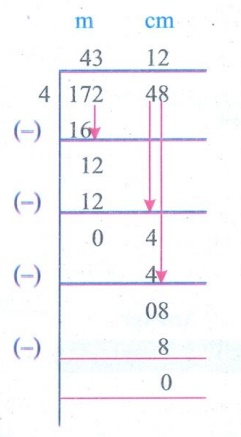Home | | Maths 5th Std | Exercise 4.1 (Length)

# Exercise 4.1 (Length)

Text Book Back Exercises Questions with Answers, Solution : 5th Maths : Term 1 Unit 4 : Measurements : Exercise 4.1 (Length)

Exercise 4.1

1. Fill in the blanks

a. 7m 5cm = 705 cm

b. 505 mm = 50 cm 5 mm

c. 326 m = 32600 cm

d. 5km 30 m = 5030 m

e. 650 cm = 6 m 50 cm

2. True or False

a) 600 m is 6 mm. (False)

b) 7000 m is 7 km.(True)

c) 400 cm is 4 km. (False)

d) 770 mm is 77 cm. (True)

e) 9000 m is 90 mm (False)

3. Find the sum of the following.

a. 17m 450cm + 52m 300cmb. 75km 400m + 37km 300m + 52km 750mc. 4cm 8mm + 5cm 9mm4. Subtract the following

a. 15km 450m − 13km 200m.b. 750m 840mm − 370m 480 mmc. 5km 400m − 3km 350m5. Multiply the following.

a. 350m 45cm × 7

b. 25km 300m × 6

c. 37m 350mm × 8

6. Divide the following:

a. 950km 800m ÷ 5b. 49m 770mm ÷ 7c. 172m 48cm ÷ 4LIFE ORIENTED PROBLEMS

a. Saravanan had chosen to drive his vehicle from puducherry to Chennai for a distance of 165 Kms. While starting his vehicle, his odometer showed 00015. What will be the reading of the odometer, When he reaches Chennai?

The reading of the odometer at the starting = 00015km +

Distance travelled                                            =     165km

When he reaches Chennai the reading of the Odometer = 00180km

b. Karthik Raja decided to travel from A. He moves 1Km east to reach B. Then he goes 2 Km towards north and reaches C. Then he goes 1 Km towards west and reaches D. If he goes 2Km towards South, Where would he reach? Draw a Diagram and Justify it. Also find out the total distance he travelled.He would reach A.

Total distance he travelled = (1 + 2 + 1 + 2)km = 6 km.

c. Sangeetha has just finished building a new house with garden area. She measured the garden area and found it to be 6m × 6m. Suppose she has to put a pole every 1m, how many poles are required? Each pole is of height 1.5m from the ground. What should be the total length of the fencing material to fence the garden?

Garden side a = 6m

Perimeter of the Garden = 4 × 6m = 24m

Poles are put every 1m except the 4 comers.

Required poles = (24−4) = 20

Height of each pole = 1.5m

Height of 20 poles = 20 × 1.5m = 30m

d. One students needs 1m 25 cm cloth to stich a shirt. What is the total length of cloth need to stitch 22 shirts?

Length of cloth needed for 1 shirts = 1 m 25cm

Length of cloth needed for 22 shirts =Length of cloth needed for 22 shirts = 27m 50cm.

e. The distance from village A to village B is 3 km 450 m. The distance from village B to village C 5 km 350 m. How long will be the road laid from village A to village C?

The distance from village A to village B = 3 Km 450 m

The distance from village B to village C = 5 Km 350 m

The distance between village A to village C = [3 Km 450 m] + [5 Km 350 m] = 8 Km 800 m

The length of the road laid from village A to village C = 8km 800m

8. Create the story problem using the pictures given below:a) A student needs 2m 50cm cloth to stitch a skirt. What is the total length of cloth needed to stitch 35 skirts.

Length of cloth needed for 1 skirt = 2m 50crn

Length of cloth needed for 35 skirts =Length of cloth needed for 35 skirts = 87m 50 cm

b) The length of 225 m 75 cm rubber tube was cut into 25 pieces. What is the length of each piece?

The length of 25 pieces of rubber tube = 225m 75cm

The length of each piece of rubber tube = 225m 75 cm ÷ 25The length of each piece of rubber tube = 9 m 03 cm

c) A carpenter cuts a long wood into 65 pieces. The length of each wooden piece is 3 m 45 cm. What is the length of the original long wood?

The length of each wooden piece = 3m 45cm

The length of 65 wooden pieces =The length of original long wood = 224 m 25cm.

d) A factory produced 4515 water pipes. It was distributed equally to 7 shops. How many water pipes were distributed to each shop.

Water pipes distributed to 7 shops = 4515

Water pipes distributed to 1 shop = 4515 ÷ 7Water pipes distributed to each shop = 645

Exercise: 4.1

1. a) 705 cm b) 50 cm 5 mm c) 32600 cm d) 5300 m e) 6m 50 m

2. a) false b) true c) false d) true e) false

3. a) 69 m 750 cm b) 165 km 450 m c) 10 cm 7 mm

4. a) 2 km 250 m b) 380 m 360 mm c) 2 km 50 m

5. a) 2453 m 15 cm b)151 km 800 m c) 298 m 800 mm

6. a) 190 km 160 m b) 7m 110 mm c) 43m 12cm

7. a) 000322 b) 6 km c) 20 poles, 30 m d) 27 m 50 cm e)8 km 800 m

Tags : Measurements | Term 1 Chapter 4 | 5th Maths , 5th Maths : Term 1 Unit 4 : Measurements
Study Material, Lecturing Notes, Assignment, Reference, Wiki description explanation, brief detail
5th Maths : Term 1 Unit 4 : Measurements : Exercise 4.1 (Length) | Measurements | Term 1 Chapter 4 | 5th Maths